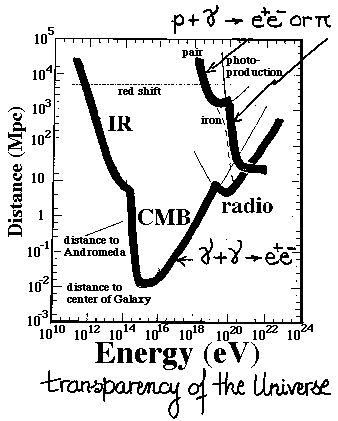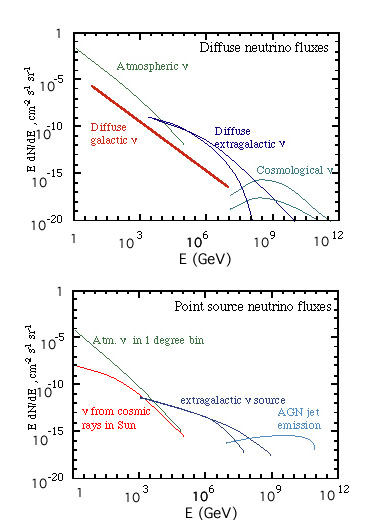## Neutrino Astrophysics

The Smoot group is active in the field of high-energy neutrino detection.

## Introduction

Why high-energy neutrino astronomy?

The reason for high-energy neutrino astronomy is to open up all wavelengths for astronomy and to peer into sources that would be opaque to photons and protons. Above GeV (roughly the rest-mass energy of a proton) energy wavelengths are less than 10^{-14} cm.

At high energies photons (gamma-rays) and protons are not viable probes.

• TeV photons are absorbed on the infrared extragalactic background. Higher energies are blocked by the cosmic microwave background and then the radio background.
• Protons with energies less than 10^19 eV do not point back to their origin because Galactic magnetic fields bend them significantly. At five times that energy (5 x 10^19 eV) they are degraded/absorbed by the cosmic radiation field because of photo-pion production and to a lesser extend because of photo-pair production.

With a high-energy neutrino detector we can open up a new window on the Universe. New windows have usually meant new discoveries.

## Scientific Motivation

The fundamental scientific motivation for high-energy neutrino astronomy is that• Neutrinos can come from cosmological distances.
• Neutrinos can escape from optically thick sources.
Thus neutrinos will allow us to observe what we cannot with other detectors. In particular we will be able to observe sources at cosmological distances. This includes both diffuse and nearly point-like sources. E.g., Based upon the GRO (Compton Gamma-Ray Observatory) full sky survey one would anticipate that there are of the order of one hundred ultra-high-energy particle sources, probably Active Galaxies (AGNs) that should be detectable with a sufficiently large detector. It is also quite likely that Gamma-Ray Bursters (GRBs) are sources of high-energy neutrinos. There is roughly one GRB per day detectable by GRO type instruments. (Over 1000 observed todate.)

A measurement of the flux, energy spectrum, angular distribution, and timing of high-energy neutrinos is a fundamental observation of the Universe.

### Neutrino Astronomy/Astrophysics

Measuring the flux is the goal but it is useful to make a rough estimate of what the diffuse and point source flux might be. The figure below show such an estimate.For more recent and detail calculations by group members see Neutrino Event Rate Estimate page

A high-energy neutrino detector will also be able to conduct a number of Particle/High Energy Physics measurements.

### Particle/High Energy Physics measurements

• Indirect Detection of WIMPs of high mass by neutrino detection
• Neutrino Oscillations
Failure to detect neutrino oscillations will set a limit on the neutrino mass difference squared of 10^{-16} eV^2, which is 5 orders of magnitude better than can be achieved using the long baseline / low energy solar neutrinos.
• The first direct detection of tau-neutrinos (if there are Neutrino Oscillations ).
• Detection of antielectron-neutrinos producing W- by the Glashow resonance at 6.3 PeV.

## Scientific Goals and Requirements on the Detector

The scientific goals/requirements for the detector to achieve outstanding scientific return are:

### Detector Scientific Requirements

• Reconstruct muon trajectories to an accuracy of 1 degree or better
• Determine particle cascade energies, direction, location, and time
• Observe a large fraction of the sky continously
It is important and straightforward for the detector to observe a large fraction of the sky simultaneously, a key for GRB observations.

## Km-scale Detector Concept

We do not detect the neutrino directly but detect its interaction and interaction products. When a neutrino interacts with a proton or neutron (or an electron) it will produce a cascade of particles. This cascade of particles will generally build up to a substantial number of particles and then die out on the scale of 10 meters. If it is a charged-current reaction,
• an electron-neutrino will produce an electron,
• a muon-neutrino will produce a muon,
• a tau-neutrino will produce an tau.
The
• electron will immediately produce an electromagnetic shower of particles,
• the muon is penetrating and will propagate great distances and emerge from the production particle cascade. A TeV muon will travel kilometers through water and rock. At TeV energies the muon will deposit energy per unit pathlength = A + B*E and B*E will be larger than A so that the energy deposited per unit length is effectively proportional to the energy of the muon.
• the tau will travel a mean distance, d = (e/m_tau c^2) c t_tau, (which is about 100 meters for a 2 PeV tau) and decay. Nearly every decay produces a mini-jet of charged particles (and pi-zero gamma-rays) containing the majority of the tau's energy and producing a hadronic/electromagnetic particle cascade.

These particles and particle cascade when in water (or ice) will produce a lot of light primarily through the Cerenkov effect. This Cherenkov light comes out as a cone-shaped shock wave at about 40-degrees from the particles' trajectory.

The detector concept is an array of optical modules (OMs) which detects the time of arrival (to roughly one nanosecond) and intensity of the Cherenkov light. By comparing the arrival timing and intensity of this light one can attempt to reconstruct the event geometry and energy deposition.

## KM3 Project

KM3 a telescope designed to detect and identify point sources of high-energy (greater than 1 TeV) neutrinos

At present a group at the University of California, specifically at LBNL (Lawrence Berkeley National Laboratory) and at the Space Sciences laboratory, and their collaborators at JPL, ... are engaged in a Research and Development Effort for a detector on the scale of a cubic kilometer (hence KM3).

### Stage I: Major R & D Thrusts

• RAND (Radio Array Neutrino Detection)
a receiver array to detect radio emission from ultra-high energy neutrino interactions in the South Polar Ice Cap.